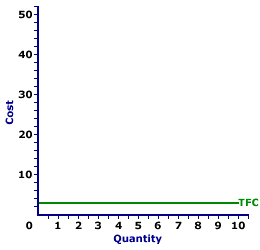Sunday  April 11, 2021
 AmosWEB means Economics with a Touch of Whimsy!AS: The abbreviaion for aggregate supply, which is the total (or aggregate) real production of final goods and services available in the domestic economy at a range of price levels, during a given time period. Aggregate supply (AS) is one half of the aggregate market analysis; the other half is aggregate demand. Aggregate supply, relates the economy's price level, measured by the GDP price deflator, and aggregate domestic production, measured by real gross domestic product. The aggregate supply relation is generally separated into long-run aggregate supply, in which all prices and wages and flexible and all markets are in equilibrium, and short-run aggregate supply, in which some prices and wage are NOT flexible and some markets are NOT in equilibrium.TOTAL FIXED COST CURVE:

A curve that graphically represents the relation between total fixed cost incurred by a firm in the short-run product of a good or service and the quantity produced. This curve is constructed to capture the relation between total fixed cost and the level of output, holding other variables, like technology and resource prices, constant. Because total fixed cost are, in fact, fixed, the total fixed cost curve is, in fact, a horizontal line. The total fixed cost curve is one of three total cost curves, the other two are total cost curve and total variable cost curve.
Total Fixed Cost CurveThe total fixed cost curve is the most straightforward of the three total curves (the other two being total cost curve and total variable cost curve). The reason for such straightforwardness is that total fixed cost is fixed. It is the same at all output levels.

The total fixed cost curve can be derived in two ways. One is to plot a schedule of numbers relating output quantity and total fixed cost. The other is to vertically subtract the total variable cost curve from the total cost curve. The total fixed cost curve can be used to derive average fixed cost.

Total fixed cost is visually illustrated by the graph to the right, which is the total fixed cost curve for the short-run production of Wacky Willy Stuffed Amigos (those cute and cuddly armadillos and tarantulas). The quantity of Stuffed Amigos production, measured on the horizontal axis, ranges from 0 to 10 and the total fixed cost incurred in the production of Stuffed Amigos, measured on the vertical axis, is \$3 at each quantity of production.

Because total fixed cost is fixed, the total fixed cost curve is a horizontal line that intersects the vertical axis at \$3. If production is 0 Stuffed Amigos, total fixed cost is \$3. If Stuffed Amigo production is 10, total fixed cost is \$3. If production of Stuffed Amigos is boosted to a billion, then total fixed cost is \$3.

 <= TOTAL FIXED COST TOTAL-MARGINAL RELATION =>Recommended Citation:

TOTAL FIXED COST CURVE, AmosWEB Encyclonomic WEB*pedia, http://www.AmosWEB.com, AmosWEB LLC, 2000-2021. [Accessed: April 11, 2021].

Check Out These Related Terms...

Or For A Little Background...

And For Further Study...
Search Again?GRAY SKITTERY[What's This?] Today, you are likely to spend a great deal of time looking for a downtown retail store looking to buy either a travel case for you toothbrush or a looseleaf notebook binder. Be on the lookout for malfunctioning pocket calculators.Your Complete ScopeDuring the American Revolution, the price of corn rose 10,000 percent, the price of wheat 14,000 percent, the price of flour 15,000 percent, and the price of beef 33,000 percent."He, who every morning plans the transactions of the day, and follows that plan, carries a thread that will guide him through a labyrinth of the most busy life."-- Victor Hugo, WriterAR(N)A nth-order Autoregressive ProcessA PEDestrian's Guide Xtra CreditTell us what you think about AmosWEB. Like what you see? Have suggestions for improvements? Let us know. Click the User Feedback link.| | | | | | | | | | |
| | | |

Thanks for visiting AmosWEB
Copyright ©2000-2021 AmosWEB*LLC
Send comments or questions to: WebMaster